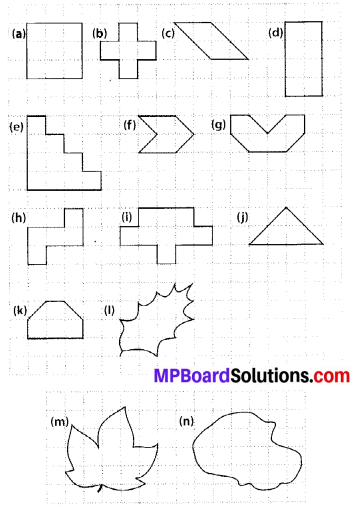# MP Board Class 6th Maths Solutions Chapter 10 Mensuration Ex 10.2

## MP Board Class 6th Maths Solutions Chapter 10 Mensuration Ex 10.2

Question 1.
Find the areas of the following figures by counting square:Solution:
(a) Number of filled squares = 9
∴ Area covered by filled squares
= (9 × 1) sq units = 9 sq units

(b) Number of filled squares = 5
∴ Area covered by filled squares
= (5 × 1) sq units = 5 sq units

(c) Number of fully-filled squares = 2
Number of half-filled squares = 4
∴ Area covered by fully-filled squares
= (2 × 1) sq units = 2 sq units
Area covered by half-filled squares
= (4 × $$\frac{1}{2}$$) sq units = 2 sq units
∴ Total area = (2 + 2) sq units = 4 sq units

(d) Number of filled squares = 8
∴ Area covered by filled squares
= (8 × 1) sq units = 8 sq units(e) Number of filled squares = 10
∴ Area covered by filled squares
= (10 × 1) sq units = 10 sq units

(f) Number of fully-filled squares = 2
Number of half-filled squares = 4
∴ Area covered by fully-filled squares
= (2 × 1) sq units = 2 sq units
Area covered by half-filled squares
= (4 × $$\frac{1}{2}$$) sq units = 2 sq units
∴ Total area = (2 + 2) sq units = 4 sq units

(g) Number of fully-filled squares = 4
Number of half-filled squares = 4
∴ Area covered by fully-filled squares
= (4 × 1) sq units = 4 sq units
Area covered by half-filled squares
= (4 × $$\frac{1}{2}$$) sq units = 2 sq units
∴ Total area = (4 + 2) sq units = 6 sq units

(h) Number of filled squares = 5 .
∴ Area covered by filled squares
= (5 × 1) sq units = 5 sq units

(i) Number of filled squares = 9
∴ Area covered by filled squares
= (9 × 1) sq units = 9 sq units

(j) Number of fully-filled squares = 2
Number of half-filled squares = 4
∴ Area covered by fully-filled squares
= (2 × 1) sq units = 2 sq units
Area covered by half-filled squares
= (4 × $$\frac{1}{2}$$) sq units = 2 sq units
∴ Total area = (2 + 2) sq units = 4 sq units(k) Number of fully-filled squares = 4
Number of half-filled squares = 2
∴ Area covered by fully-filled squares
= (4 × 1) sq units = 4 sq units
Area covered by half-filled squares
= (2 × $$\frac{1}{2}$$) sq units = 1 sq units
∴ Total area = (4 + 1) sq units = 5 sq units

(l) Number of fully-filled squares = 3,
Number of half-filled squares = 2,
Number of more than half-filled squares = 4
and number of less than half-filled squares = 4.
Now, estimated area covered by
fully-filled squares = 3 sq units,
half-filled squares = (2 × $$\frac{1}{2}$$) sq units
= 1 sq unit,
more than half-filled squares = 4 sq units
and less than half-filled squares
= 0 sq unit
∴ Total area = (3 + 1 + 4 + 0) sq units
= 8 sq units.(m) Number of fully-filled squares = 7,
Number of more than half-filled squares = 7
and number of less than half-filled squares = 5
Estimated area covered by
fully-filled squares = 7 sq units,
more than half-filled squares = 7 sq units
and less than half-filled squares = 0 sq unit
∴ Total area = (7 + 7 + 0) sq units = 14 sq units

(n) Number of fully-filled squares = 10,
Number of more than half-filled squares = 8
and number of less than half-filled squares = 5
Estimated area covered by
fully-filled squares = 10 sq units,
more than half-filled squares = 8 sq units
less than half-filled squares = 0 sq unit
∴ Total area = (10 + 8 + 0) sq units
= 18 sq units.